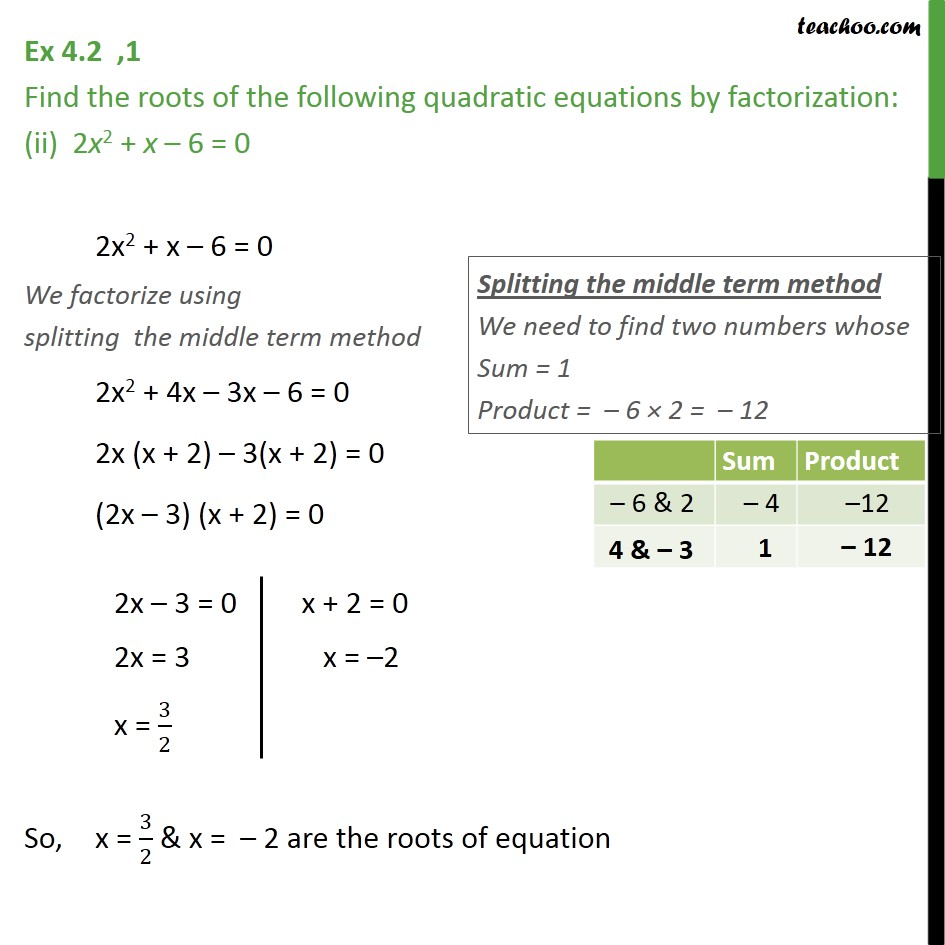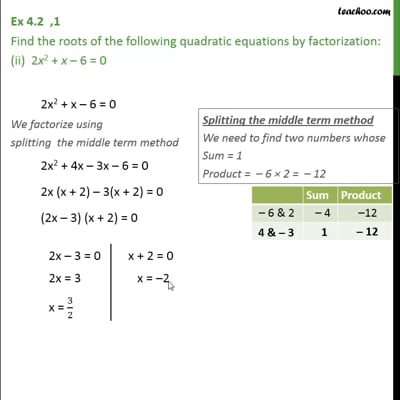Ex 4.2

Chapter 4 Class 10 Quadratic Equations
Serial order wiseThis video is only available for Teachoo black users

Introducing your new favourite teacher - Teachoo Black, at only ₹83 per month

### Transcript

Ex 4.2 ,1 Find the roots of the following quadratic equations by factorization: (ii) 2x2 + x 6 = 0 2x2 + x 6 = 0 We factorize using splitting the middle term method 2x2 + 4x 3x 6 = 0 2x (x + 2) 3(x + 2) = 0 (2x 3) (x + 2) = 0 So, x = 3/2 & x = 2 are the roots of equation